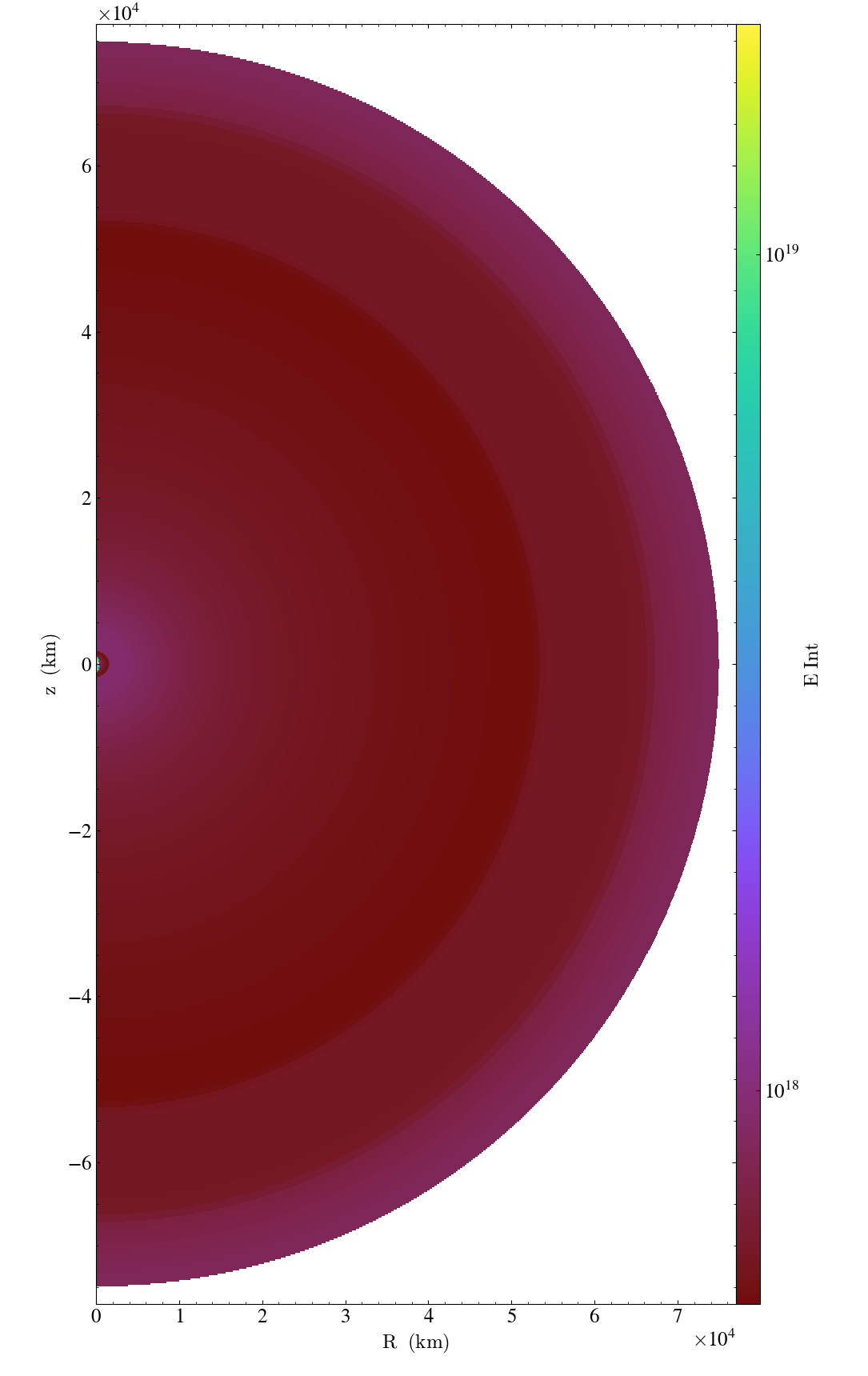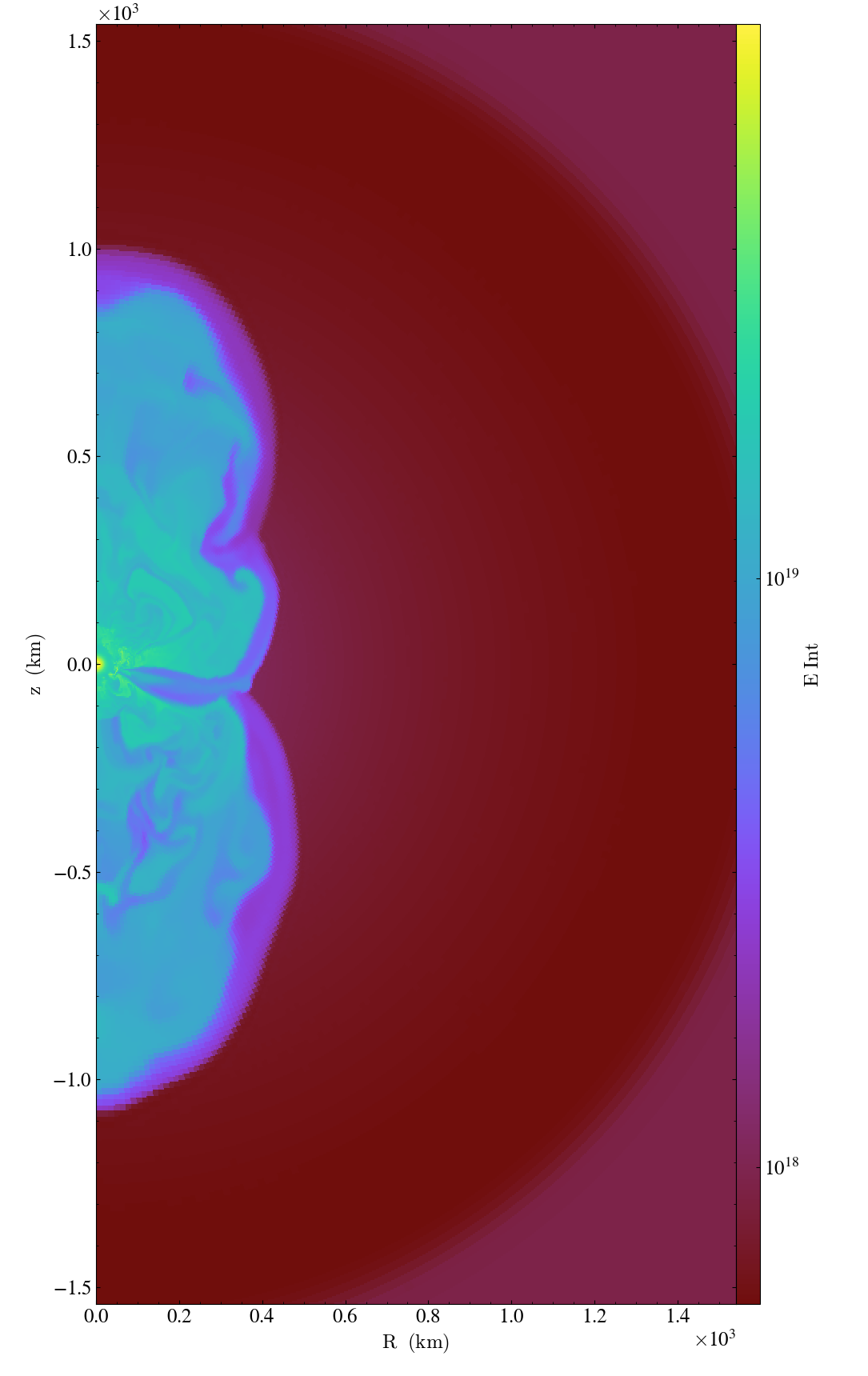For all the data formats that yt knows about, reading a dataset is supposed to be trivial – the yt.load command knows how to parse the metadata of something like 30-50 different dialects of data, generate the in-memory representation, and even manage things like the coordinate system (Spherical? Cartesian? etc) and how to apply spatial transformations.

But, if you’re just experimenting or trying to get a new frontend going, right now it’s too hard to load data into yt. (Before you think I’m passing the buck, though: this is kind of my fault.)

Last week, I worked with some folks to load in data from a simulation code called CHIMERA. The data specifically was a 2D axially symmetric dataset, with log-spaced bins in the radial direction. The file format was really quite straightforward, too!

Usually when developing a frontend to make all of this easier, the first step is to load the data into yt using the Stream frontend. This has functions like load_amr_grids, load_uniform_grid, load_unstructured_mesh, load_particles and so on. But, it was pretty annoying to load the data into yt. And it seems like it should be a lot simpler.

Let’s dig in, following along as I had explored it.

import h5py
import yt
import numpy as np

f = h5py.File("chimera_000661800_grid_1_01.h5", "r")
f.keys()

<KeysViewHDF5 ['abundance', 'analysis', 'fluid', 'mesh', 'metadata', 'radiation', 'tracking']>


Without any additional information, my first guess is to look at the mesh dataset – that’s likely where I’m going to find the information for how the dataset is organized.

f["/mesh"].keys()

<KeysViewHDF5 ['array_dimensions', 'cycle', 'd_omega', 'dx_cf', 'dy_cf', 'dz_cf', 'i_frame', 'last_frame', 'my_hyperslab_group', 'nz_hyperslabs', 'ongrid_mask', 'phi_index_bound', 'r_comv', 'radial_index_bound', 't_bounce', 'theta_index_bound', 'time', 'time_steps', 'x_cf', 'x_ef', 'y_cf', 'y_ef', 'z_cf', 'z_ef']>


Well, here we go! Lots of interesting things to look at. I’ll save a bit of time here and note that the things we’re interested in are the array_dimensions and the various _ef arrays: x_ef, y_ef, z_ef. These are the dimensions of the simulation and the cell edges, respectively. There are other interesting things here as well, but for our current purposes, this is what we want to look at.

dims = f["/mesh/array_dimensions"][()]
xgrid, ygrid, zgrid = (f["/mesh/{}_ef".format(ax)][()] for ax in 'xyz')

xgrid.shape, ygrid.shape, zgrid.shape, dims

((723,), (241,), (2,), array([722, 240,   1], dtype=int32))


We’re dealing with cell edges here so we have one more in each dimension than the cell-centered variables. Great! Everything is going according to plan so far.

(You might be thinking to yourself, “Gee, wouldn’t it be nice if this 1:1 mapping of values to what yt expects was easier to set up?” I certainly was.)

Let’s see what fields we can load up:

f["/fluid"].keys()

<KeysViewHDF5 ['LScompress', 'agr_c', 'agr_e', 'c_eos_i', 'dimeos', 'dudt_nu', 'dudt_nuc', 'e_int', 'entropy', 'eos_rho', 'grav_x_c', 'grav_y_c', 'grav_z_c', 'press', 'rho_c', 't_c', 'u_c', 'v_c', 'v_csound', 'wBVMD', 'w_c', 'ye_c', 'ylep']>


Some might have reduced dimensionality, so we’ll take a look at what will be the most obvious to load in by identifying which have the right dimensionality.

import collections
collections.Counter(f["/fluid"][v].shape for v in f["/fluid"])

Counter({(): 1, (722,): 1, (723,): 1, (1,): 2, (2,): 1, (1, 240, 722): 17})


Looks like the fluid fields are stored in reverse order from the dims array, so let’s populate our dictionary (all in-memory) with this info.

We’ll grab the transpose, though, so that we keep the same ijk ordering as specified in the dimensions.

data = {n: v[()].T for n, v in f["/fluid"].items() if v.shape == tuple(dims[::-1])}
data.keys()

dict_keys(['dudt_nu', 'dudt_nuc', 'e_int', 'entropy', 'grav_x_c', 'grav_y_c', 'grav_z_c', 'press', 'rho_c', 't_c', 'u_c', 'v_c', 'v_csound', 'wBVMD', 'w_c', 'ye_c', 'ylep'])


We can now proceed! Let’s generate our semi-structured mesh from our coordinate info and load it all in.

This next function generates a full set of coordinates and connectivity to make our hexahedral mesh look like a structured system. (This is a place that yt could use some improvement, to speed things up by not requiring this hoop-jumping!)

coord, conn = yt.hexahedral_connectivity(xgrid, ygrid, zgrid)


Next up will be loading things in using the yt.load_hexahedral_mesh function, so let’s look at the docstring for that.

yt.load_hexahedral_mesh?

Signature:
data,
connectivity,
coordinates,
length_unit=None,
bbox=None,
sim_time=0.0,
mass_unit=None,
time_unit=None,
velocity_unit=None,
magnetic_unit=None,
periodicity=(True, True, True),
geometry='cartesian',
unit_system='cgs',
)
Docstring:
Load a hexahedral mesh of data into yt as a
:class:~yt.frontends.stream.data_structures.StreamHandler.

This should allow a semistructured grid of data to be loaded directly into
yt and analyzed as would any others.  This comes with several caveats:

* Units will be incorrect unless the data has already been converted to
cgs.
* Some functions may behave oddly, and parallelism will be
disappointing or non-existent in most cases.
* Particles may be difficult to integrate.

Particle fields are detected as one-dimensional fields. The number of particles
is set by the "number_of_particles" key in data.

Parameters
----------
data : dict
This is a dict of numpy arrays, where the keys are the field names.
There must only be one. Note that the data in the numpy arrays should
define the cell-averaged value for of the quantity in in the hexahedral
cell.
connectivity : array_like
This should be of size (N,8) where N is the number of zones.
coordinates : array_like
This should be of size (M,3) where M is the number of vertices
indicated in the connectivity matrix.
bbox : array_like (xdim:zdim, LE:RE), optional
Size of computational domain in units of the length unit.
sim_time : float, optional
The simulation time in seconds
mass_unit : string
Unit to use for masses.  Defaults to unitless.
time_unit : string
Unit to use for times.  Defaults to unitless.
velocity_unit : string
Unit to use for velocities.  Defaults to unitless.
magnetic_unit : string
Unit to use for magnetic fields. Defaults to unitless.
periodicity : tuple of booleans
Determines whether the data will be treated as periodic along
each axis
geometry : string or tuple
"cartesian", "cylindrical", "polar", "spherical", "geographic" or
"spectral_cube".  Optionally, a tuple can be provided to specify the
axis ordering -- for instance, to specify that the axis ordering should
be z, x, y, this would be: ("cartesian", ("z", "x", "y")).  The same
can be done for other coordinates, for instance:
("spherical", ("theta", "phi", "r")).
File:      ~/yt/yt/yt/frontends/stream/data_structures.py
Type:      function


Looks like we’ve got just about everything except for the bounding box. So let’s generate that.

bbox = np.array([
[xgrid.min(), xgrid.max()],
[ygrid.min(), ygrid.max()],
[zgrid.min(), zgrid.max()]
])
bbox

array([[0.00000000e+00, 7.70275059e+09],
[0.00000000e+00, 3.14159265e+00],
[0.00000000e+00, 6.28318531e+00]])


And the last thing we need to do is to specify the geometry. This dataset is spherical (but with only one value along the azimuthal direction, in this case) and so we have to specify which coordinate is which. We do that using the geometry keyword argument, where we specify not only the geometry’s name, but the axis-ordering.

Here we have:

• r is the first dimension
• theta is the declination, which spans an interval of $\pi$, so it’s the second dimension
• phi is our azimuthal angle, which goes $2\pi$ (and here is identical at all azimuthal angles) so it’s the third.
ds = yt.load_hexahedral_mesh(data, conn, coord, bbox = bbox, geometry = ("spherical", ('r', 'theta', 'phi')))

s = yt.SlicePlot(ds, "phi", "e_int")
s.show()Looks kinda right! Unfortunately it’s a bit tricky to navigate these coordinates in the version of yt I’m running, so we have to do a bit of work to get ourselves zoomed in to see things closer up.

s.set_center( (0.0, 0.0) )
s.zoom(50)
s.pan_rel((0.5, 0.0))We’re now at the point that things can be used, but … it was kind of irritating getting here!

## What have we learned?

Good question! The first thing I thought as I was going through this was that I did an awful lot of work for what amounted to a one-to-one mapping between the dataset and the arguments that yt expected. It feels like I should just be able to mark all of this up with some metadata to make the loading process much easier, before writing a specific frontend that manages this all for us.

Over the last few days, a collaborator (Sam Walkow) at UIUC and I started brainstorming what a metadata schema would look like that could map from a file format directly to yt. One of the advantages of having this explicitly typed and validated is that we can check for errors earlier in the process and we can also make logical leaps from one piece of information to another.

For instance, note that above we define the bbox independently of the coordinate system. But, for a dataset like this, they will usually be the same! And, since we’re dealing with an HDF5 dataset, we should be able to just specify that.

We’ve started drafting what this might look like, using JSON-Schema via PyDantic and some helper classes. Ideally, we should be able to specify something in yaml that describes how to get everything we want. Imagine, if instead of what we showed above, we had a small schema that yt could read and that could potentially even live as a sidecar file! (And then we could even register a mimetype for display in Jupyterlab…) We’ve been exploring using this for declarative analysis, to reduce the burden of writing out lots of boilerplate code, but it seems like it would be a great match for this, too.

Anyway, I was speculating that something like this – but accounting for an explicitly declared list of fields, etc – might be sufficient.

metadata:
filetype: hdf5
geometry:
dimensions: /mesh/array_dimensions
spherical:
order: [r, theta, phi]
bbox: implicit
hexahedral_mesh:
edges: [/mesh/x_ef, /mesh/y_ef, /mesh/z_ef]
fields:
implicit: true
root: /fluid
validate: true
process: transpose


This doesn’t yet work, but I think it gives a target for how we might think about making it much easier to load in data in formats that have such a nice mapping between yt and the dataset. And it leaves enough room for things like on-demand loading (which the stream frontend does support, but in an opaque way) that it could be quite nice to extend this in the future.

I’ve pretty much talked myself into doing something like this and seeing how it’d work. Maybe it could be a SciPy project next week!

## Acknowledgments

Thanks to Bronson Messer, Ronan Hix and Samantha Walkow for the data and speculating, and to Nathan Goldbaum for planting this “let’s just write a yaml declaration” idea in my head a million years ago. (It took me a while to catch up to how insightful an idea that was.)

It’s also probably worth mentioning that these amazing revelations I’m having are … not that new to lots of people using data! I mean, folks working on things like wcsaxes and netCDF metadata and lots of others have trod this ground before!##### Matthew Turk
###### Assistant Professor of Information Sciences

I am interested in the intersection of data analysis, visualization and open source in the sciences.